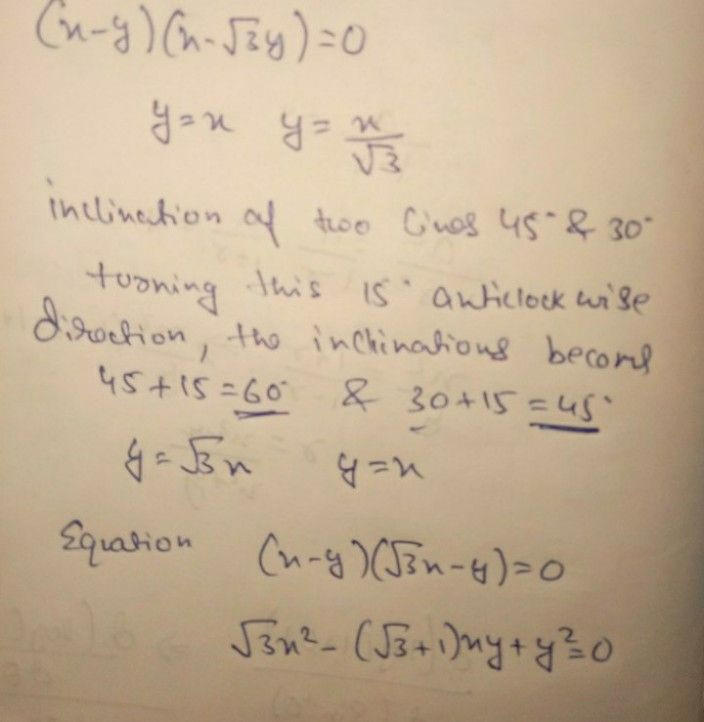Symbol
Problem$3.$ If the function $f\left(x\right)=\left(\dfrac {x^{2}-1} {x-1}$ $k$ when $x≠1$ when $x=1$ is given to be continuous at $x=1,$ then the value of $k$ is
10th-13th grade
English
SolutionQanda teacher - shivaramakif uncleared please do ask again